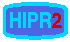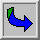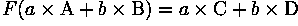# Non-linear Filtering

Suppose that an image processing operator F acting on two input images A and B produces output images C and D respectively. If the operator F is linear, thenwhere a and b are constants. In practice, this means that each pixel in the output of a linear operator is the weighted sum of a set of pixels in the input image.

By contrast, non-linear operators are all the other operators. For example, the threshold operator is non-linear, because individually, corresponding pixels in the two images A and B may be below the threshold, whereas the pixel obtained by adding A and B may be above threshold. Similarly, an absolute value operation is non-linear:as is the exponential operator:.